Model Specification

For a two-level response, the probability that the lesser response occurs is modeled by the probit equation as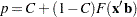The probability of the other (complementary) event is.

For a multilevel response with outcomes labeledfor, the probability,, of observing level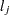is as follows: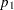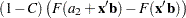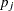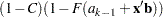Thus, for a-level response, there areadditional parameters,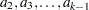, estimated. These parameters are denoted by Intercept,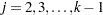, in the output.

An intercept parameter is always added to the set of independent variables as the first term in the model unless the NOINT option is specified in the MODEL statement. If a classification variable taking onlevels is used as one of the independent variables, a set ofindicator variables is generated to model the effect of this variable. Because of the presence of the intercept term, there are at most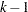degrees of freedom for this effect in the model.